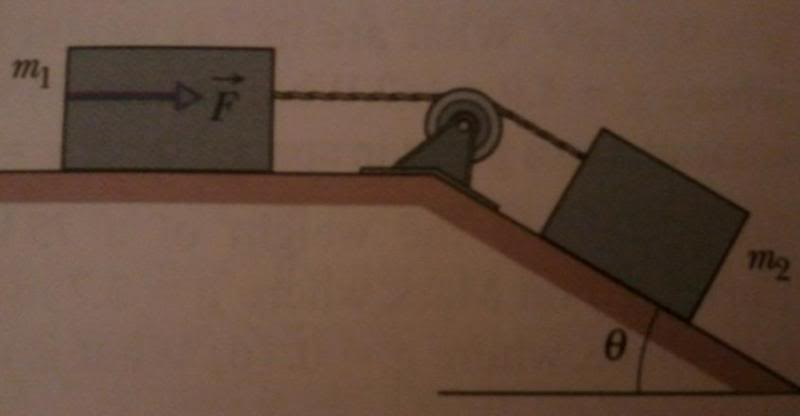# What is the maximum force allowed in this tension problem?

• Theorγ

## Homework Statement

A box (m2) with mass 1.0 kg is on a frictionless plane inclined at angle θ = 30o. It is connected by a cord of negligible mass to a box (m1) with mass 3.0 kg on a horizontal frictionless surface. Box m1 is pushed with force F. What is the maximum value of F allowed so that the cord does not slack?Fnet = ma

## The Attempt at a Solution

I first tried to find the acceleration of the system without the influence of Fp, which turned out to be 1.225 m/s2. Then I reasoned that if the boxes are moving at that speed, initiated by the m2 from gravity, then that means that F + T (tension) has to equal m1a, with an "a" larger than 1.225 m/s2.

Thus, I worked with that equation, which lead to the following:
F + T = m1a
F = m1a - T

T = 3.1 N

F = (3.0 kg)(1.225 m/s2) - 3.1 N
F = 0.575 N

So does this mean that the maximum force allowed is 0.575 N?

Last edited:
When the cord is on the verge of going 'slack', its tension is 0. Try that approach. Your answer is not correct.

When the cord is on the verge of going 'slack', its tension is 0. Try that approach. Your answer is not correct.
So does that mean that the maximum push force should be 3.1 N? Since without any force, the tension resulting from the second block going down the ramp is 3.1 N, but if the push force provides that 3.1 N, then doesn't that mean that the tension would be neutralized?

Freee Body Diagrams are essential. Draw a free body diagram of each block. What are the forces acting on each block with T=0? Then apply Newton's laws to each block, to solve for the 2 equations with 2 unknowns, F and a.

Suppose that m2 (on the slope) were allowed to slide all by itself (no rope connected). How fast would it accelerate?

Now the rope is connected between the masses. How should mass m1 (on the flat) move in order to allow mass m2 to continue to accelerate at its 'natural' rate? That is, no tension in the rope.

Freee Body Diagrams are essential. Draw a free body diagram of each block. What are the forces acting on each block with T=0? Then apply Newton's laws to each block, to solve for the 2 equations with 2 unknowns, F and a.

Forces on Block 1
m1a = F + T
m1a = F + 0

Forces on Block 2
m2a = Fgx2 - T
m2a = Fgx2 - 0
a = Fgx2/m2

m1a = F
m1(Fgx2/m2) = F
F = 14.7 N?

Suppose that m2 (on the slope) were allowed to slide all by itself (no rope connected). How fast would it accelerate?

Now the rope is connected between the masses. How should mass m1 (on the flat) move in order to allow mass m2 to continue to accelerate at its 'natural' rate? That is, no tension in the rope.

The second block should naturally accelerate at 4.9 m/s2, without tension slowing it down.